# 1 Introduction

Time-series clustering is affected by several factors, such as the characteristics of time-series themselves, the choice of distance or centroid function, etc. In many situations, run-time characteristics are more important, e.g. when the amount of memory is limited by the system, or when excessive running times must be avoided. Most of these aspects cannot be generalized, especially in regards to evaluation of correctness, but things like complexity or growth rate of an algorithm can be assessed relatively more easily. To get an idea of the running times that can be expected for some of the algorithms included in `dtwclust`, a series of timing experiments were made. Said experiments were not concerned with correctness or accuracy, only with timing characteristics.

The experiments were run with `R` v3.6.0 and `dtwclust` v5.5.3. The `microbenchmark` package (v1.4.6) was also used for most of the experiments. The computer used was running GNU/Linux (LTS kernel v4.19) with an `i5-6500` Intel processor (4 cores) and 16GB of RAM. The whole set of experiments took approximately 22 hours to complete. The data used comes from the Character Trajectories set (Lichman 2013), which have different lengths and are originally multivariate series with 3 variables; the univariate versions were extracted from these. All scripts are available online on GitHub. Naturally, since we are dealing with execution times, the experiments cannot be reproduced exactly, but hopefully the median times would not vary too much between systems with similar characteristics.

# 2 Distance experiments

## 2.1 Calculations for single time-series

First we look at the results of the timing experiments for single time-series, i.e., for distances calculated between two individual time-series. The distances tested are those included in the package. Here we look at the effect of window sizes and series’ lengths. Each calculation was repeated 500 times and the median value was extracted. Note that the vertical scales differ for the following figures.

### 2.1.1 DTW lower bounds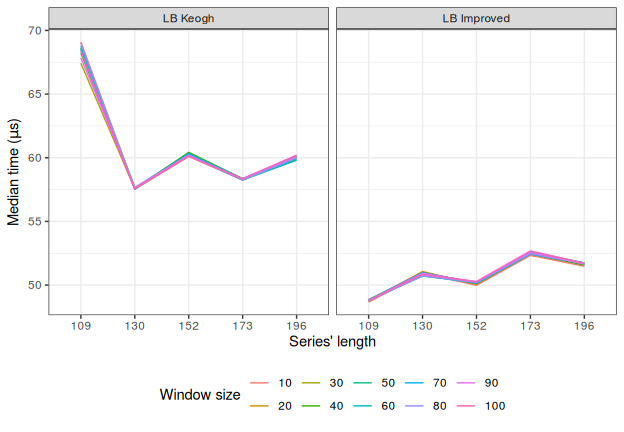The first interesting result relates to the DTW lower bounds: `lb_keogh` and `lb_improved`. The window size does not seem to have a very significant effect, and the running time appears to (mostly?) grow slowly with the series’ length. However, `lb_improved` was faster that `lb_keogh`. Considering that `lb_improved` first needs to calculate `lb_keogh` and then perform additional calculations, this is somewhat puzzling, and the reason is not immediately evident. Perhaps there are compiler optimizations at play.

### 2.1.2 Shape-based distance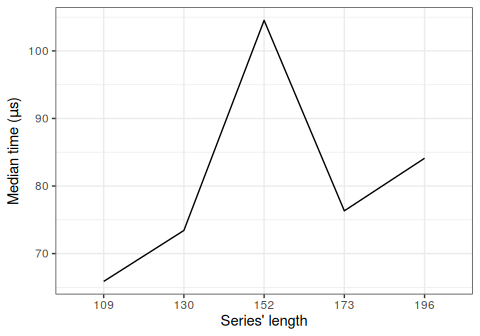The shape-based distance also presents weird behavior. While it was expected that its running time would increase with the series’ length, the bump for the length of 152 is considerably large. It is true that SBD is based on the FFT, and thus it adjusts the input series’ lengths to powers of 2, but if that were the cause, then the bump should have occurred for the series with length of 130, since the next power of 2 for 109 is 128, and it jumps to 256 for a length of 130.

### 2.1.3 DTW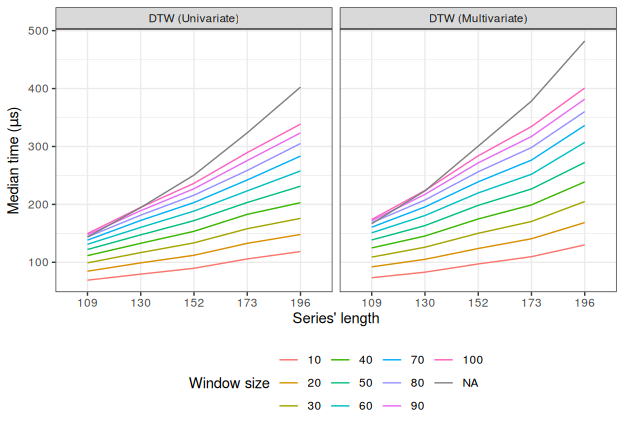In the case of DTW, we can see that a window constraint can indeed have a very significant effect on running time, considering that a window size of 10 resulted in a calculation that was about 4 times faster than when using no constraint. In this case, using multivariate series (with 3 variables) did not have a very significant effect.

### 2.1.4 Soft-DTW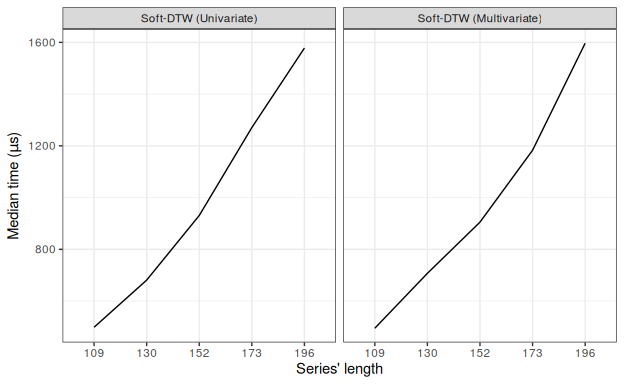In principle, the soft-DTW algorithm is very similar to that of unconstrained DTW. However, its run-time characteristics are clearly different, resulting in considerably slower calculations. Interestingly, the multivariate case was marginally faster.

### 2.1.5 Triangular global alignment kernel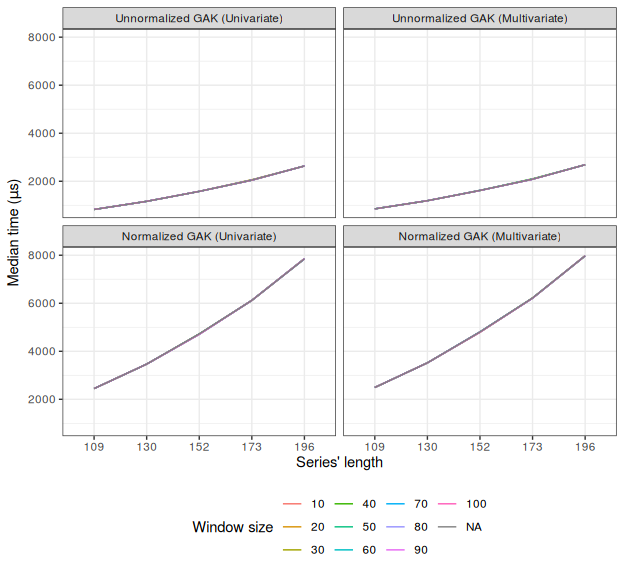The behavior of GAK was rather surprising. Its running times increase very fast with the series’ length, and neither window size nor number of variables seem to have any effect whatsoever. The normalized version is 3 times slower because it effectively calculates a GAK distance 3 times: one time for `x` against `y`, one time for `x` alone, and one time for `y` alone; these 3 values are used to calculate the normalized version.

## 2.2 Calculations for several time-series

Computing cross-distance matrices for time-series can be optimized in different ways depending on the distance that is used. In the following sections, we look at the way the included distances are optimized, and evaluate the way it affects their running times when doing distance calculations between several time-series.

These experiments were repeated 30 times and the median value was computed. We look at the effect of several factors: the length of the series, a window size where applicable, the effect of parallelization, and the size of the cross-distance matrix.

### 2.2.1 DTW lower bounds

First we assess the distances that involve the DTW lower bounds. In the following figure, the `x` axis contains the total number of cells in the cross-distance matrix, but the color is mapped to the number of columns in said matrix. This is relevant in this case because of the envelopes that need to be computed when calculating the lower bounds. These are computed for the series in `y`, which end up across the columns of the distance matrix. The applied optimization consists in calculating the envelopes for `y` only once, and re-using them across `x`. In the case of `dtw_lb` (which is based on `lb_improved`), this is also important because nearest neighbors are searched row-wise by default, and more series in `y` equates to more neighbors to consider. Also note that the `dtw_lb` calculations make more sense when the series in `x` and `y` are different (if the series are the same, the nearest neighbor of a series is always itself, so no iterations take place), which is why there are less data points in those experiments. Given the results of the previous section, the window size value was fixed at 50 for these experiments.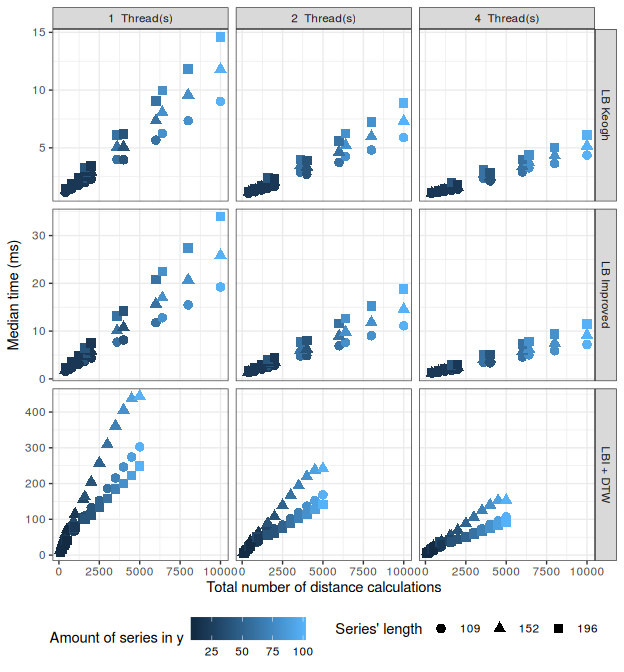The facets’ columns indicate the number of parallel threads, whereas the rows indicate the distance that was used. The vertical scales are different for each row, but they are all in milliseconds.

For `lb_keogh` and `lb_improved`, we see that the effect of the series’ length is more consistent when calculating cross-distance matrices. Parallelization with multi-threading yields better performance in a proportional way, and this time `lb_improved` was about twice as slow as `lb_keogh`. As expected, increasing the number of series in `y` increases the running times.

The behavior of `dtw_lb` is also consistent, but the length of the series affected running times in a strange way, since the calculations with series of length 152 were the slowest ones. Using multi-threading can also be advantageous in this case, and this is applied on two occasions: when estimating the initial distance matrix with `lb_improved`, and when updating the nearest neighbors’ with DTW. Nevertheless, the procedure is much slower than the lower bounds alone.

### 2.2.2 Shape-based distance

As mentioned before, the shape-based distance is based on the FFT. Similarly to the DTW lower bounds, the optimization applied here consists in calculating the FFTs only once, although in this case they must be calculated for both `x` and `y`. The results are summarized in the next figure.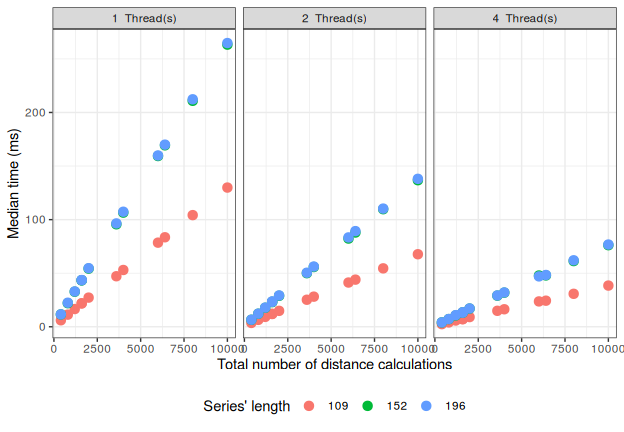The facets’ columns indicate the number of parallel threads.

For sequential calculations, we see here the expected effect of the series’ lengths. Adjusting them to powers of 2 for the FFT meant that the calculations were faster for series of length 109, and for series of length 152 and 196 the times were virtually the same (so much that the points overlap). Parallelization helped reduce times proportionally.

### 2.2.3 DTW

The DTW distance doesn’t allow for many optimizations. The version implemented in `dtw_basic` for cross-distance matrices uses less RAM by saving only 2 columns of the local cost matrix (LCM) at all times (since no back-tracking is performed in this case). Moreover, this LCM is only allocated once in each thread. The results are shown in the next figure.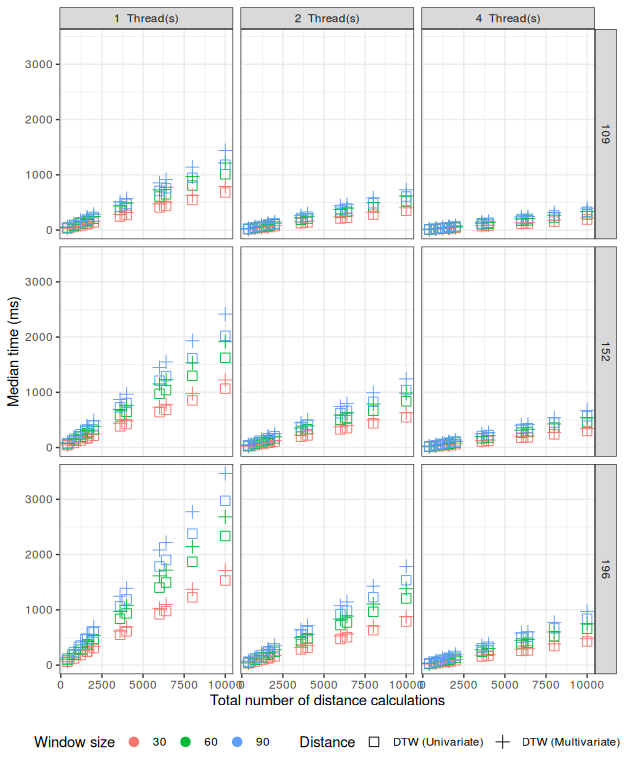The facets’ columns indicate the number of parallel threads, whereas the rows indicate the length of the series being considered (all series had the same length for each case). All times are in milliseconds.

The DTW distance presents a much more constant behavior across the board. The difference between univariate and multivariate series is very small, but window sizes and series lengths can have a very significant effect, especially for sequential calculations. Additionally, DTW benefits linearly from parallelization, since using 2 threads reduced times in half, and using 4 reduced them practically by a factor of 4. Also note that the growth is very linear, which indicates that DTW cannot be easily optimized much more, something which was already pointed out before (Ratanamahatana and Keogh 2004).

### 2.2.4 Soft-DTW

As with DTW, soft-DTW has few optimizations: its helper matrix is allocated only once in each thread during the calculation. In this case, we look only at the univariate version.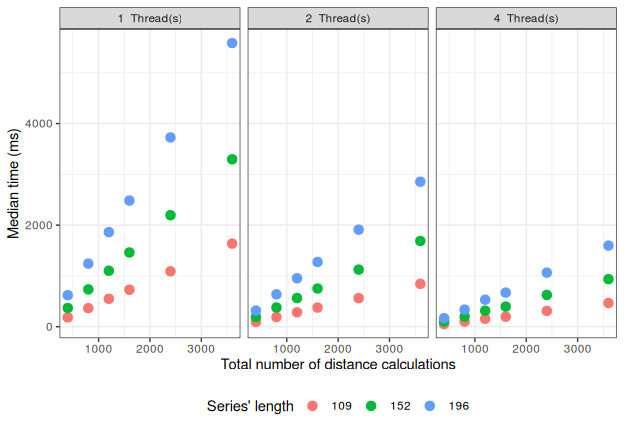The facets’ columns indicate the number of parallel threads. All times are in milliseconds.

The benefits of parallelization are also very evident for soft-DTW, and present similar characteristics to the ones obtained for DTW. Unfortunately, running times also grow very fast with the series’ length.

### 2.2.5 Triangular global alignment kernel

Finally we look at the computations with the GAK distance. As shown in the previous section, this distance is considerably slower. Moreover, only the normalized version can be used as a distance measure (as opposed to a similarity), so only the normalized version was tested.

There are 2 optimizations in place here. As mentioned before, normalization effectively requires calculating a GAK for `x` and `y` by themselves, so in order to avoid repeated calculations, these normalization factors are only computed once for cross-distance matrices. GAK also uses a helper matrix to save logarithms during the intermediate calculations, and this matrix is allocated only once in each thread.

It is worth pointing out that, in principle, the GAK code is very similar to that of DTW. However, GAK relies on logarithms, whereas DTW only uses arithmetic operations. This means that, unfortunately, GAK probably cannot be optimized much more.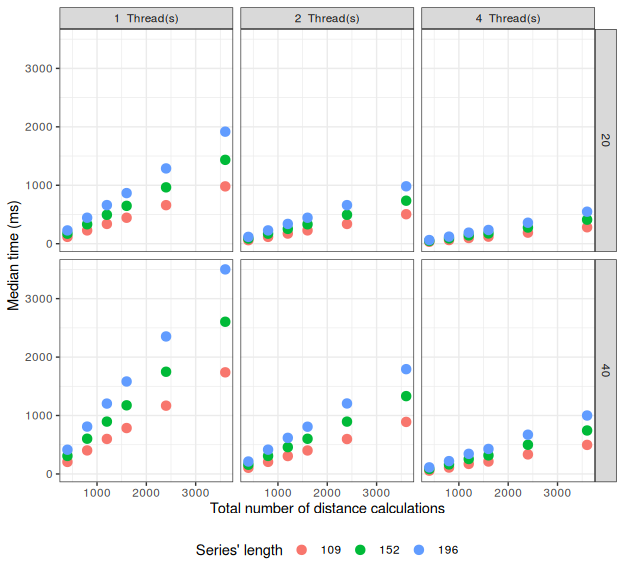The facets’ columns indicate the number of parallel threads, whereas the rows indicate the window size that was used. All times are in milliseconds.

Here we see a clear effect of window sizes and series’ lengths and, as was the case for DTW, parallelization can be very beneficial for GAK.

# 3 Prototyping experiments

In this section, we briefly look at the running times of 3 centroid functions: shape extraction, DBA and the soft-DTW procedure. Each experiment was run 30 times and the median value was computed.

## 3.1 Shape extraction

Shape extraction is based on SBD, and thus has no window constraints. The multivariate version simply applies the univariate algorithm to each of the variables in the series. It does not use parallelization right now.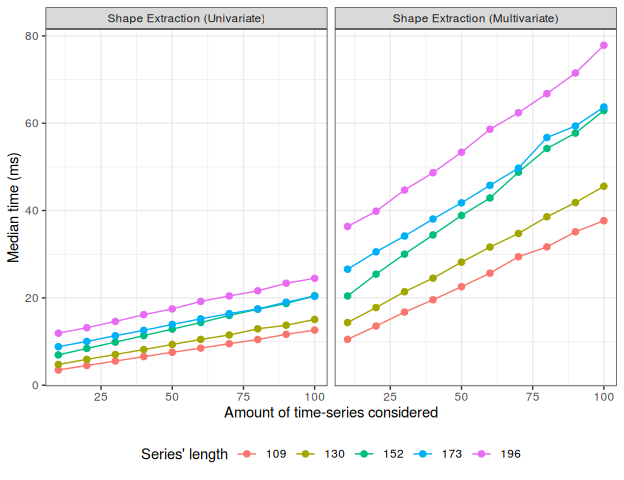As expected, the multivariate version is proportionally slower than the univariate version. The length of the series can indeed have a significant effect, and using more series naturally increases running times, albeit linearly.

## 3.2 DTW barycenter averaging

DBA is based on DTW, so it can use window constraints. Additionally, it supports multivariate series for the same reason, but in 2 variations. One variation simply uses the same strategy as shape extraction: it applies the univariate version to each of the variables and binds the resulting series. However, DBA uses the backtracking feature of DTW, and this can be computed based on an LCM calculated from two multivariate series as a whole. In `dtwclust`, the former variation is the `by-variable` version, and the latter is the `by-series` version. The implementation also supports multi-threading, separating the input series onto different threads. Also note that this implementations of DBA allocate the LCM used for backtracking only once in each thread; it does not matter if series have different lengths, as long as the LCM’s dimensions are defined based on the longest series.